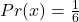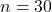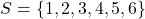Suppose you roll a cube 30 times, what is a to describe the theoretical of rolling a 1, 4, 6, 7 Please help me!

Question

Suppose you roll a cube 30 times, what is a to describe the theoretical of rolling a 1, 4, 6, 7 Please help me!

in progress 0
5 months 2021-08-19T09:14:55+00:00 1 Answers 2 views 0Step-by-step explanation:

Given— number of rolls

Required

The theoretical probability of rolling: (a) 1    (b) 4    (c) 6    (d) 7

Assume the cube is 6 sided.

The sample space is:And the probability of each is:Irrespective of the number of rolls, the theoretical probability of (a). (b), (c) and (d) is: